# Module 5: CONDITIONAL STATEMENTS

## Learning Objectives

After reading this lesson, you should be able to:

• use the if-elseif statement to address multiple conditional cases,

• identify when to use multiple if-end statements,

• program an if-elseif-else statement,

• identify the differences between elseif and else.

## What is the if-elseif statement?

An elseif conditional clause is the third and last type of conditional clause we cover. An elseif clause does essentially the same thing as an if statement since it has its own condition: except it will only be evaluated when its parent (and previous) if statement is false (see Example 1). The elseif clauses can also have multiple conditional expressions just like we discussed in Lesson 5.2 for if statements.

### Example 1

Given a real number num, write a MATLAB program that displays, “The number is positive” if the input number is positive, or “The number is negative” if the input number is negative, or “The number is zero” if the input number is zero. Run and test your program three times, using the values of num of -4.5, 0, and 6.

Solution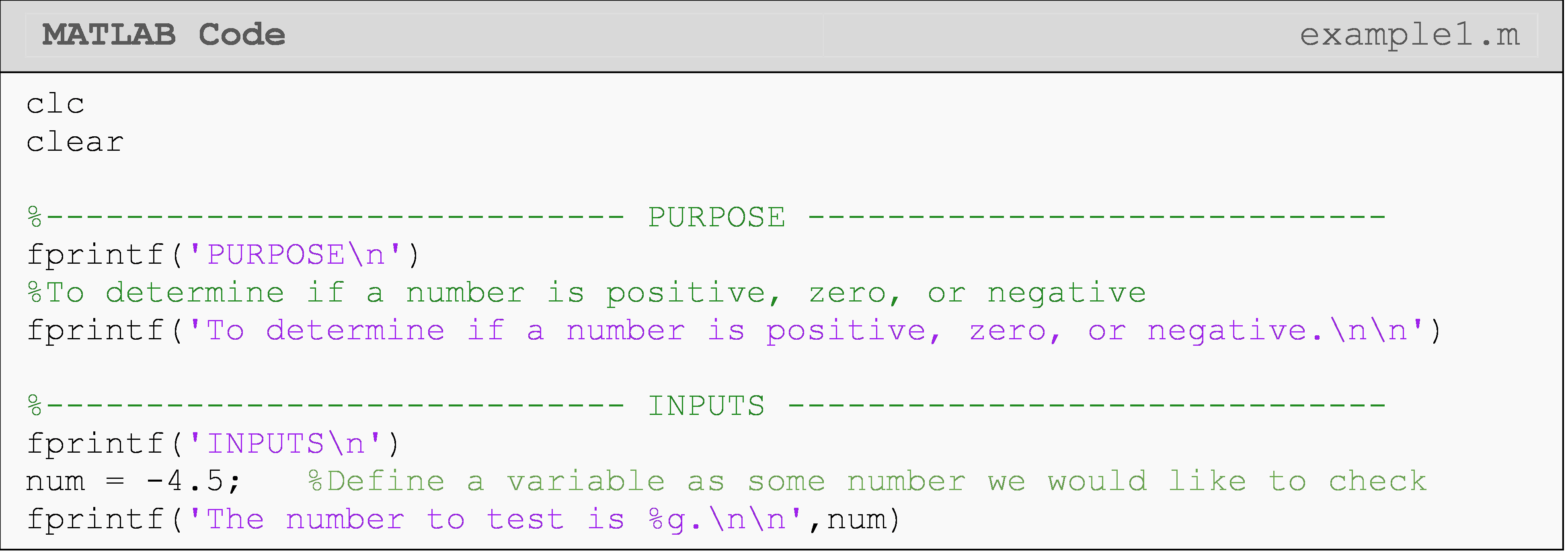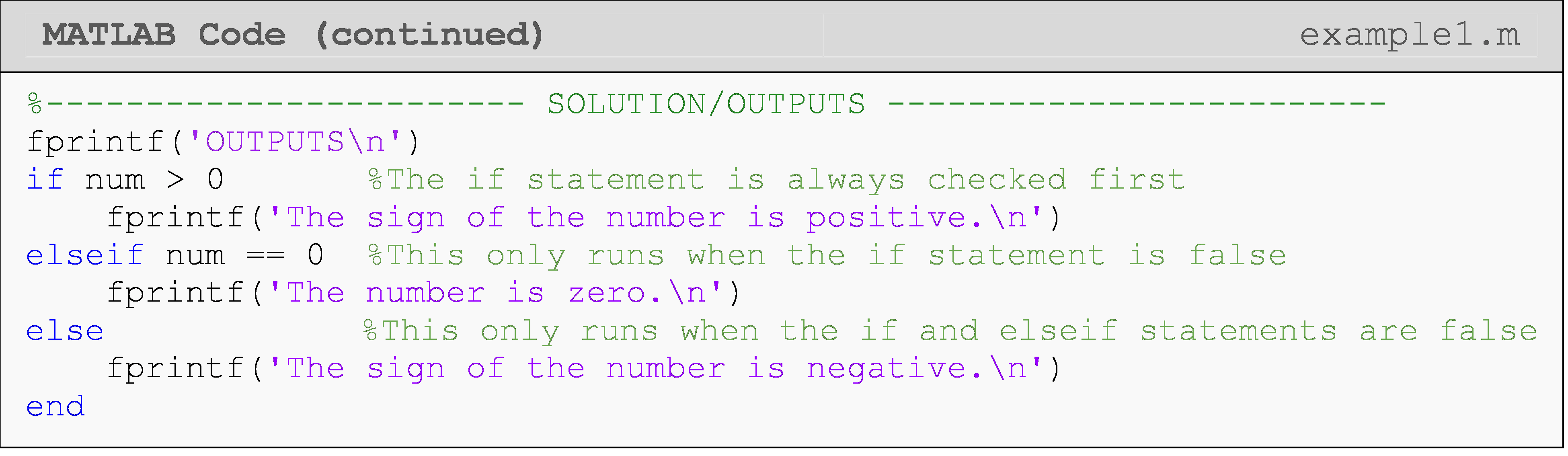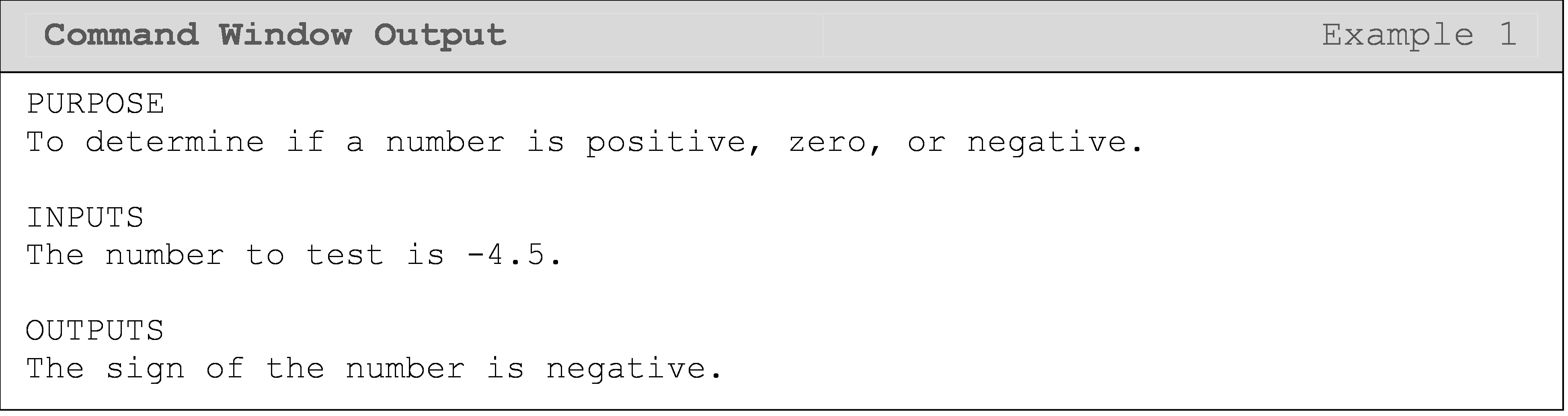Only one condition (if or elseif) will be true at once. It is also essential to note that the whole linked conditional statement is terminated by a single end.

Example 2 illustrates another use of the if-elseif statement. Here, we want to check for a certain string and conditionally run our block of code based on that. We use a special function strcmp() to avoid a “matrix dimension mismatch” error from MATLAB when comparing strings.

### Example 2

Provide a greeting based on a username entered by a string. Display the result to the Command Window.

Solution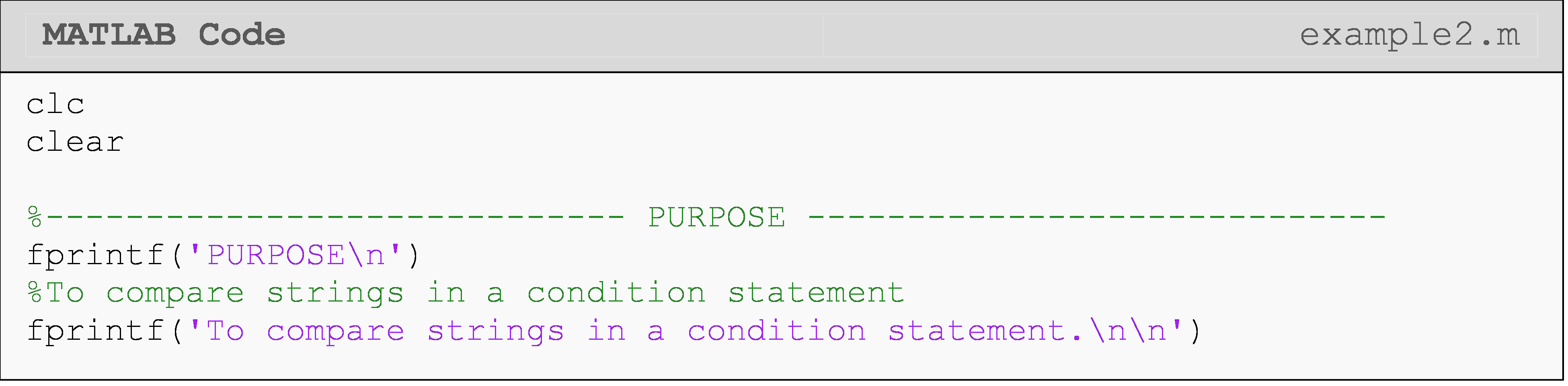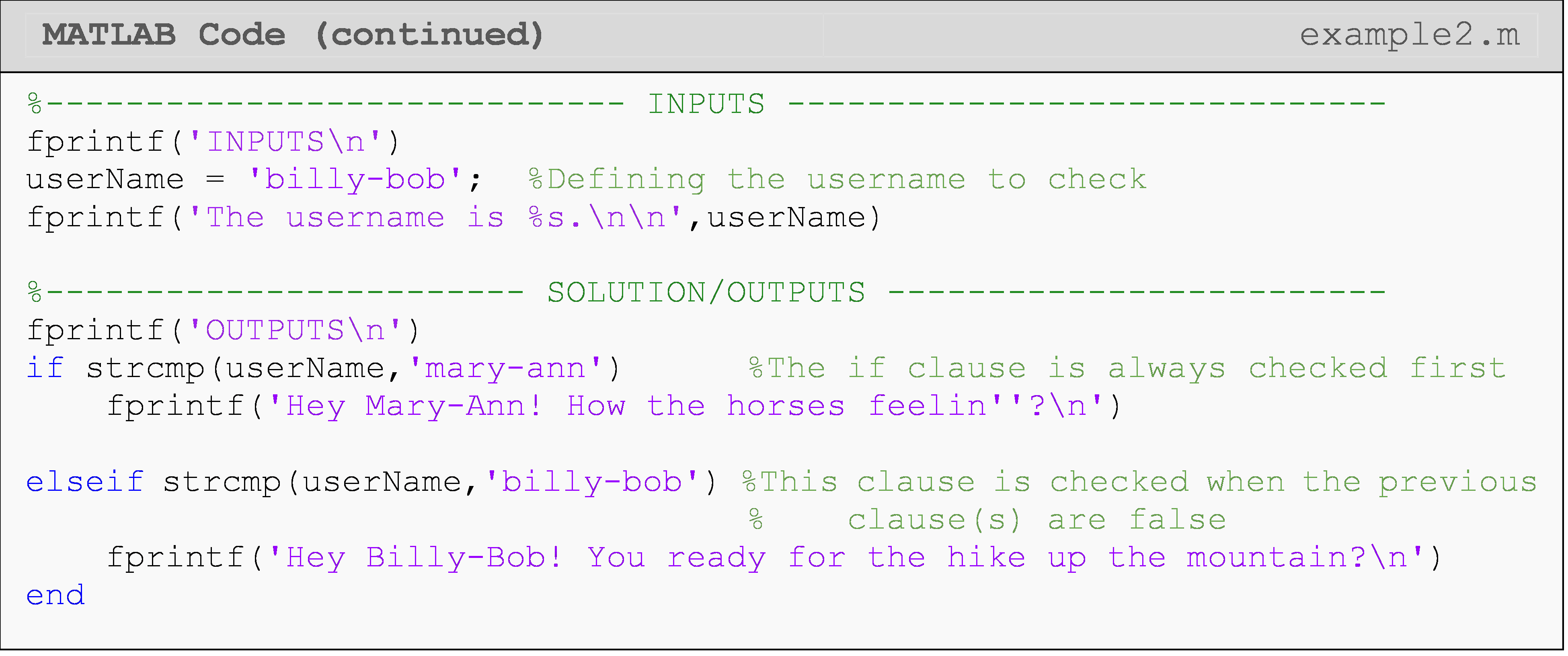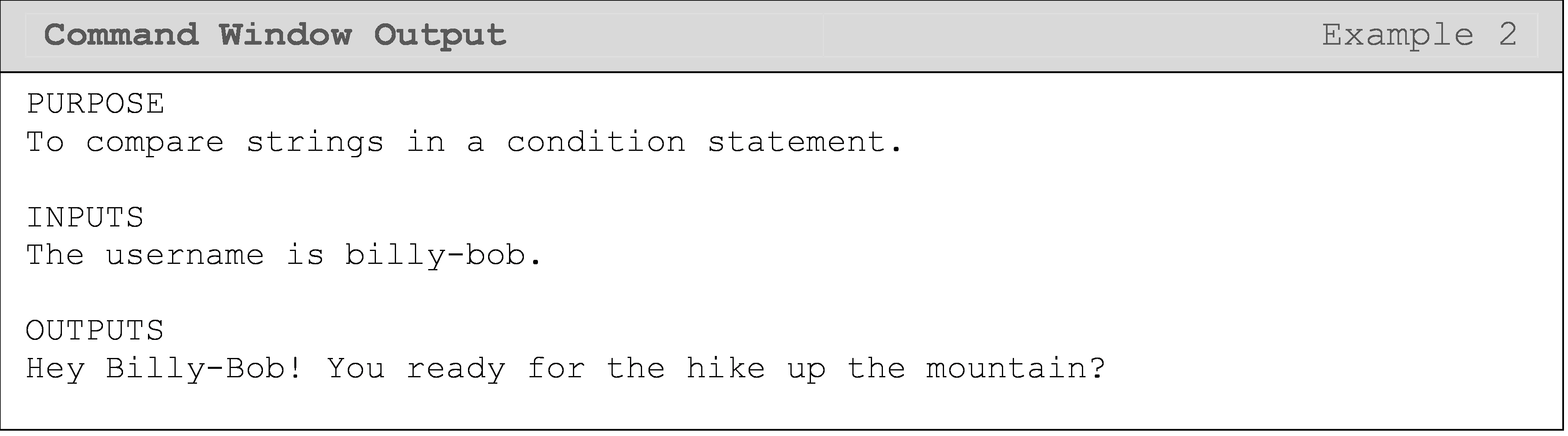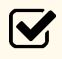Important Note: In an if-elseif-else statement, only the if OR the elseif OR the else runs.

## Independent vs. Dependent Cases

In this context, a conditional case just means that we wish to consider a specific scenario, which is described by one or more individual conditions. Independent cases can all be true simultaneously (or independently). They do not depend on each other to be true or false (see Example 3). Independent cases should be implemented with separate if statements. For example, the width and height of a beam are independent conditions (neglecting application requirements).

Dependent cases cannot be true simultaneously and should be implemented with elseif/else clauses (see Example 4). Take Example 1, for instance. These are dependent cases because only one can be true for a given input. Another example is checking the value of the cross-sectional area of a beam. The area cannot be two values at once, so checking whether the area is X or Y should be implemented as dependent cases.

### Example 3

Check whether the two independent variables x and y are within given bounds. The value of x should be less than 5, and that of y should be greater than 20. Display the result to the Command Window.

Solution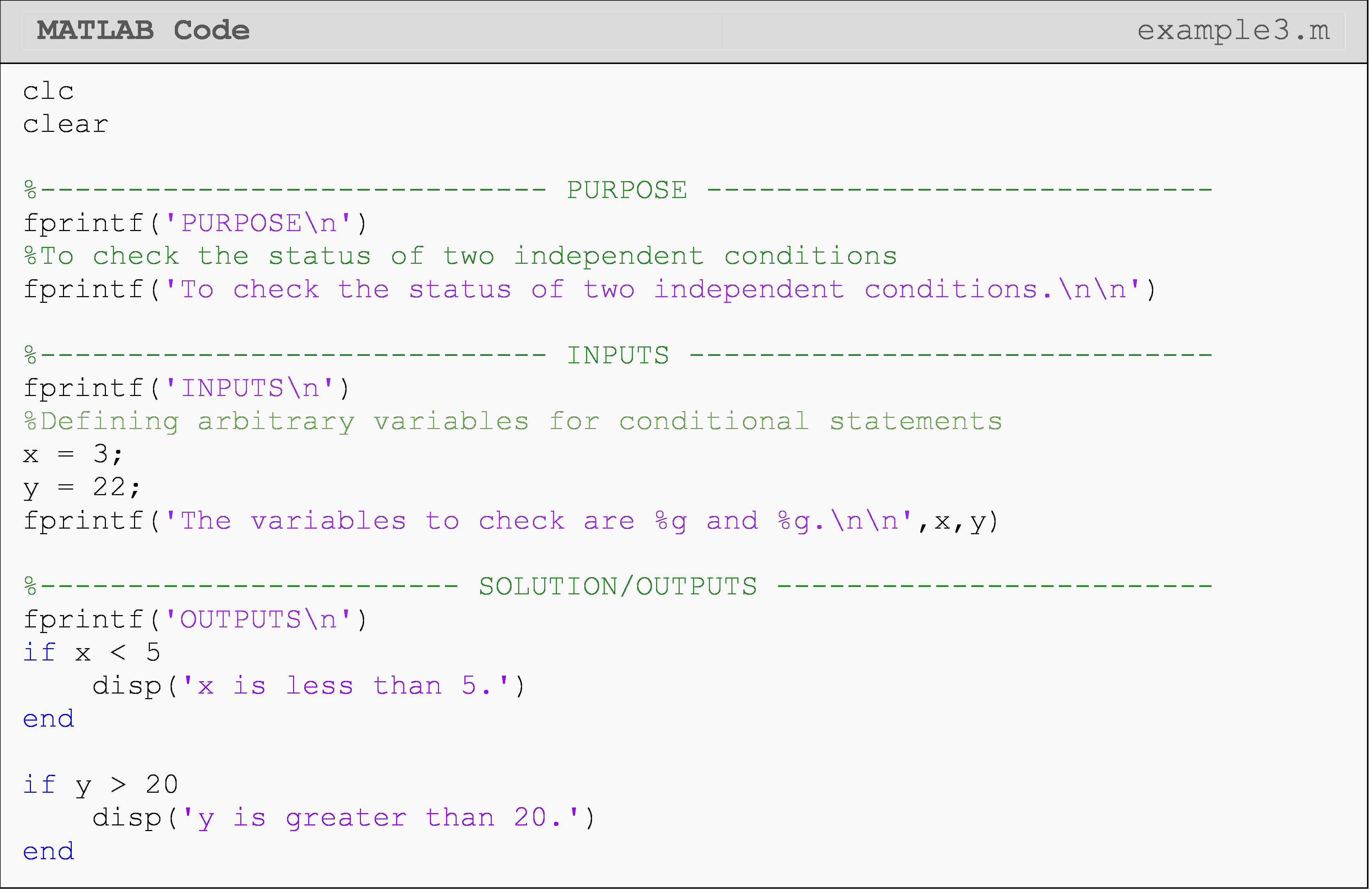Combining these if-end statements as an if-elseif statement (if x<5 and elseif y>20) would only result in the x message displaying (with the current values of x and y). You should try this for yourself!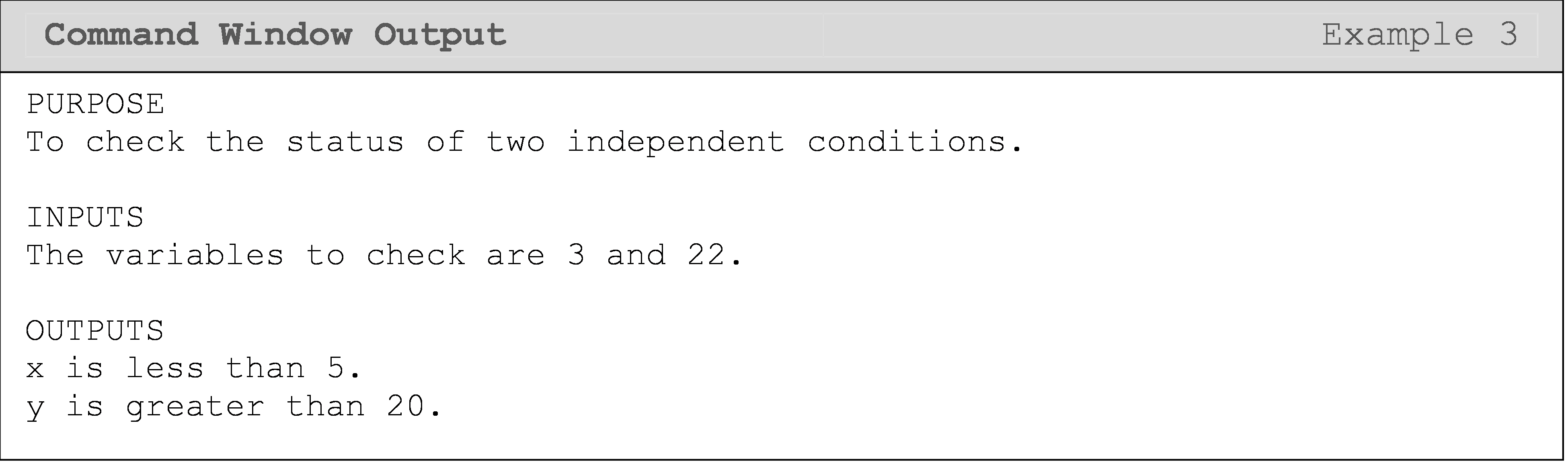In Example 4, both conditions (cases) depend on a, so they are dependent. The variable a cannot be two values simultaneously.

### Example 4

Check whether the variable a is less than five or not. Display the result to the Command Window.

Solution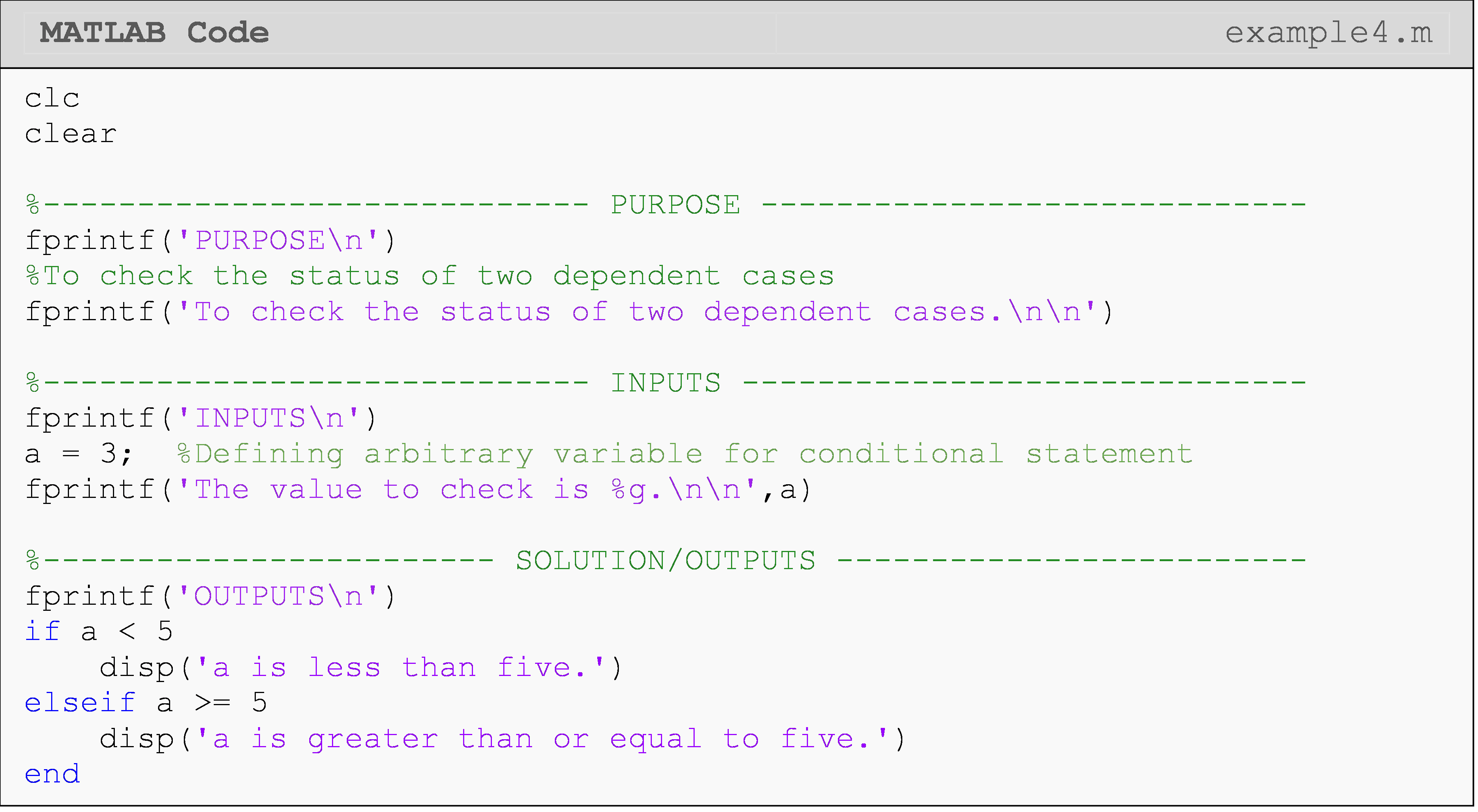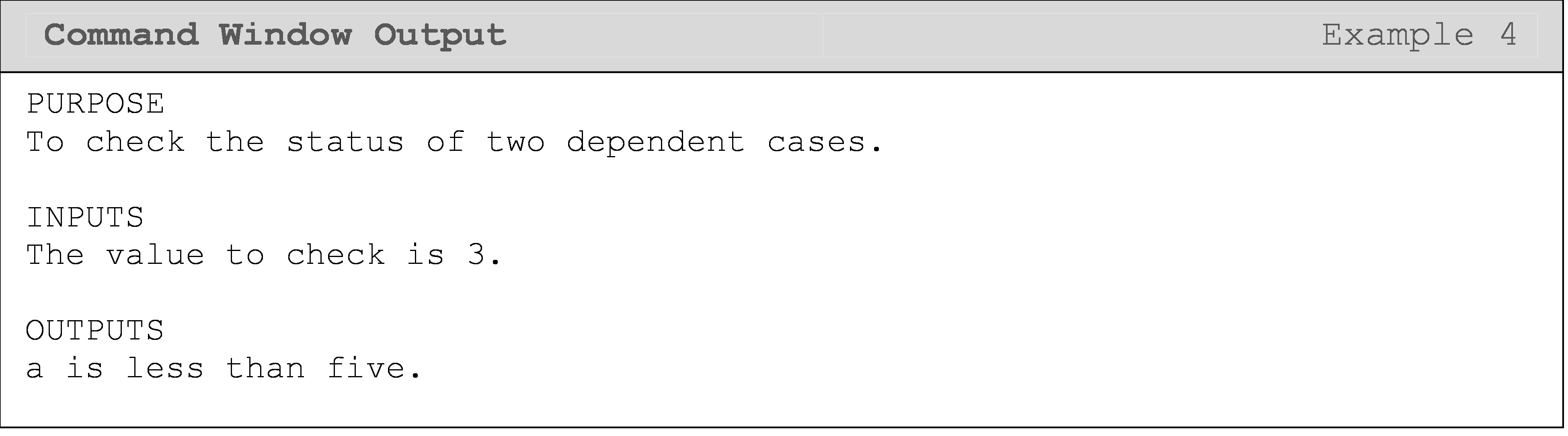If you are still unsure about the differences between dependent and independent conditions, just take a step back when you are programming and think whether the two (or more) scenarios you are considering can be true simultaneously in the “real world”. Can a = 2 AND a = 3? No! You are implementing “real world” scenarios when you program, so you should first know what is possible or not in the real world. Then write a program structure that implements it in your code.

## What is the if-elseif-else statement?

We can put everything we have learned together with an if-elseif-else statement. As you can see in Example 5, else must always come last! That is, if all previous conditions are false, the code block under the else statement will be executed.

### Example 5

Monitor the temperature of an oven. Write a program that determines whether the oven is still heating up, over the target temperature, or at the target temperature. The two necessary temperature values are given as follows. The last temperature reading from the temperature sensor of the oven is 400°F. The bake temperature set by the user is 425°F.

Solution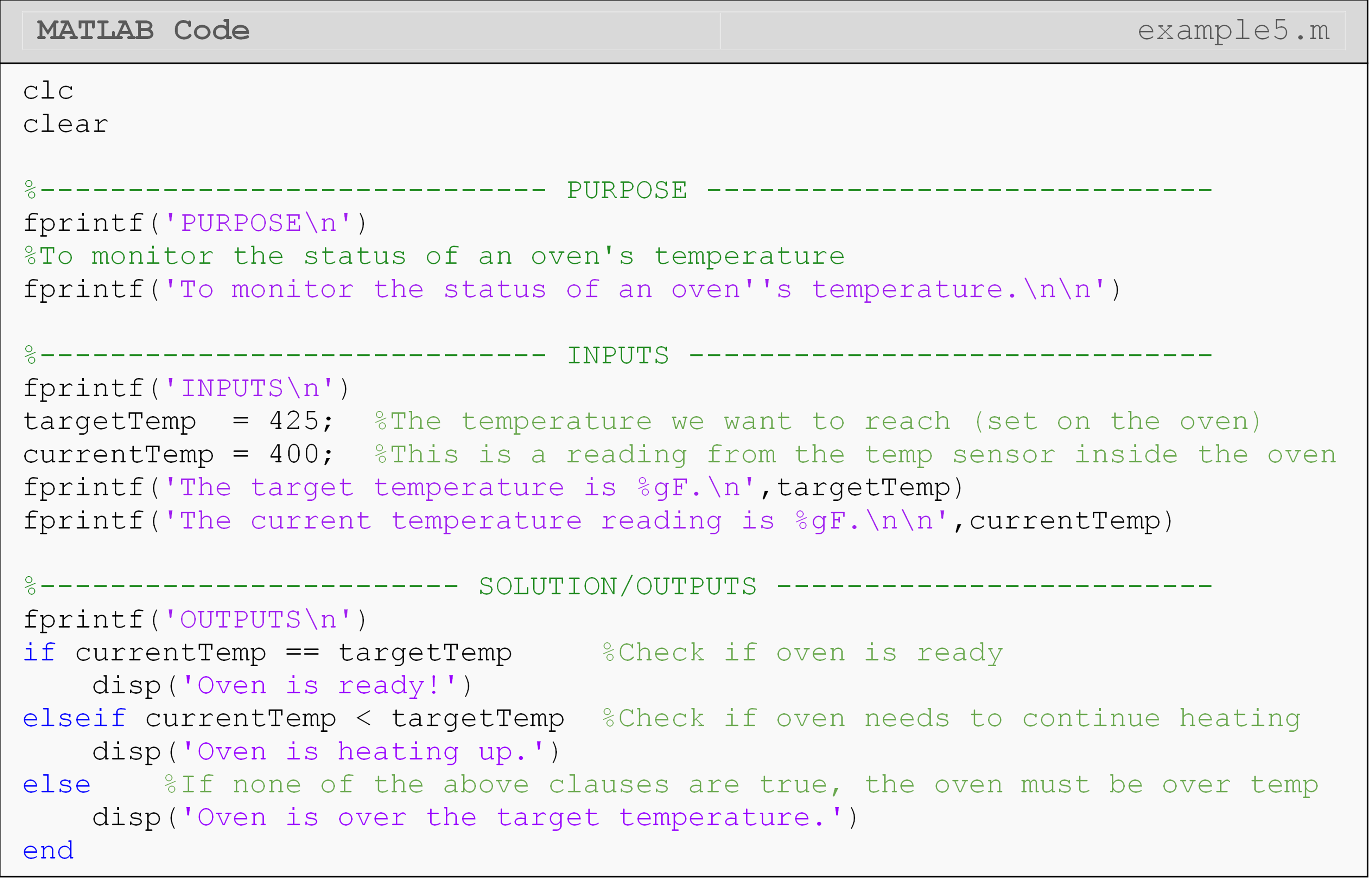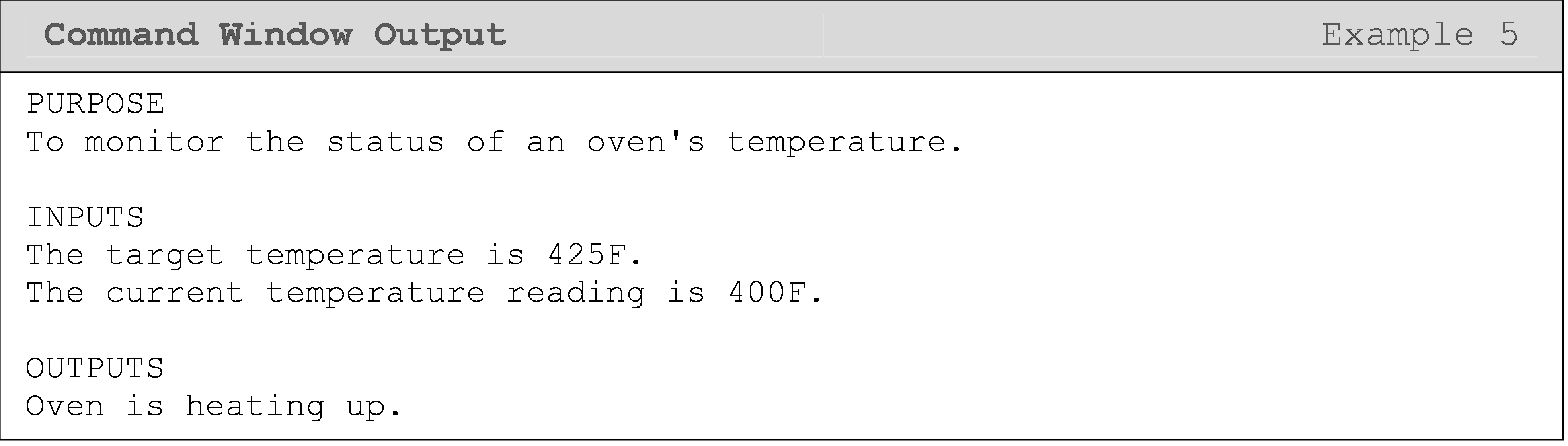Notice these are dependent cases (there is only one temperature value at a given time), so the cases are implemented with an if-elseif-else statement. Using an if-elseif-elseif statement would also yield a correctly functioning program, but it would be slightly less computationally efficient since there would be an extra condition to check in the second elseif clause.

## What is the difference between the else and elseif conditional clauses?

An elseif has its own condition, whereas else has no condition and will always run given that all conditional clauses (if or elseif) before it are false. Therefore, you should only use an else when you want to address all other possibilities. Use elseif when you want to address specific cases only, and when you want to address multiple specific cases that are unique (i.e., the cases cannot be true at the same time: a > 0 and a < 0).Important Note: else must always come last in a conditional statement.

## Lesson Summary of New Syntax and Programming Tools

 Task Syntax Example Usage Conditionally execute blocks of code in multiple exclusive areas elseif if a<5; disp('case 1'); elseif a>8; disp('case2'); end

## Multiple Choice Quiz

(1). Which of the following statements is not true about the elseif clause?

(a)  elseif must always be used in conjunction with an if statement.

(b)  elseif must have its own condition.

(c)  An elseif clause is not always executed.

(d)  One may only use up to three elseif clauses in a single if-end statement.

(2). What will the Command Window output of the following program be?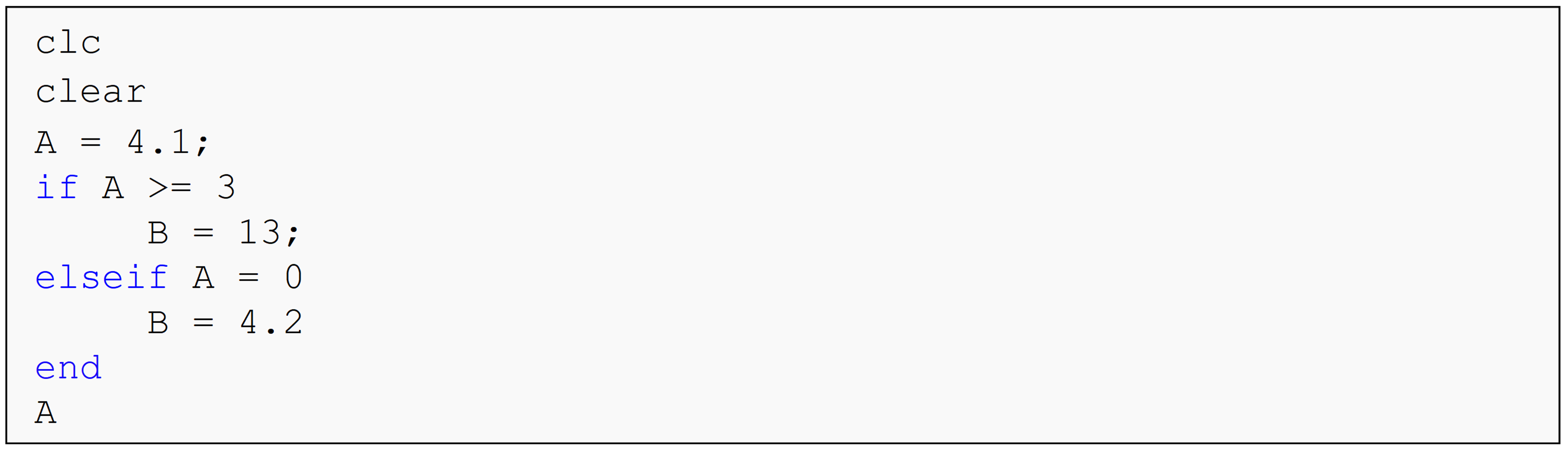(a)  A = 4.1

(b)  B = 13

(c)  Incorrect use of '=' operator.

(d)  A = 3

(3). What is the Command Window output of the following program?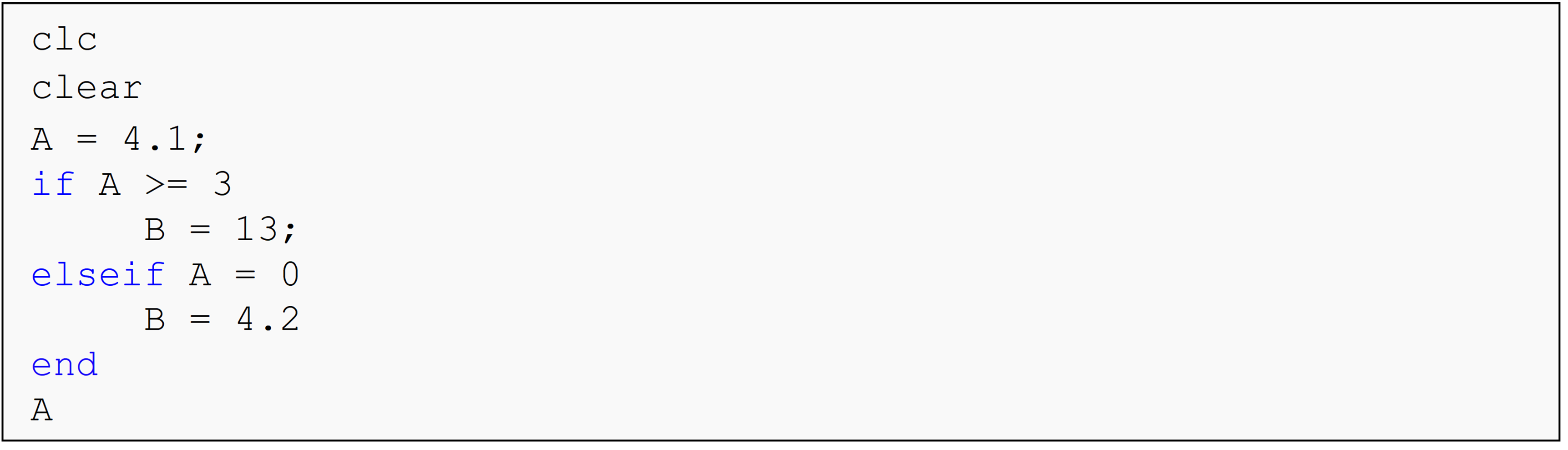(a)  Case A

(b)  Case B

(c)  Case C

(d)  Case D

(4). What is the Command Window output of the following program?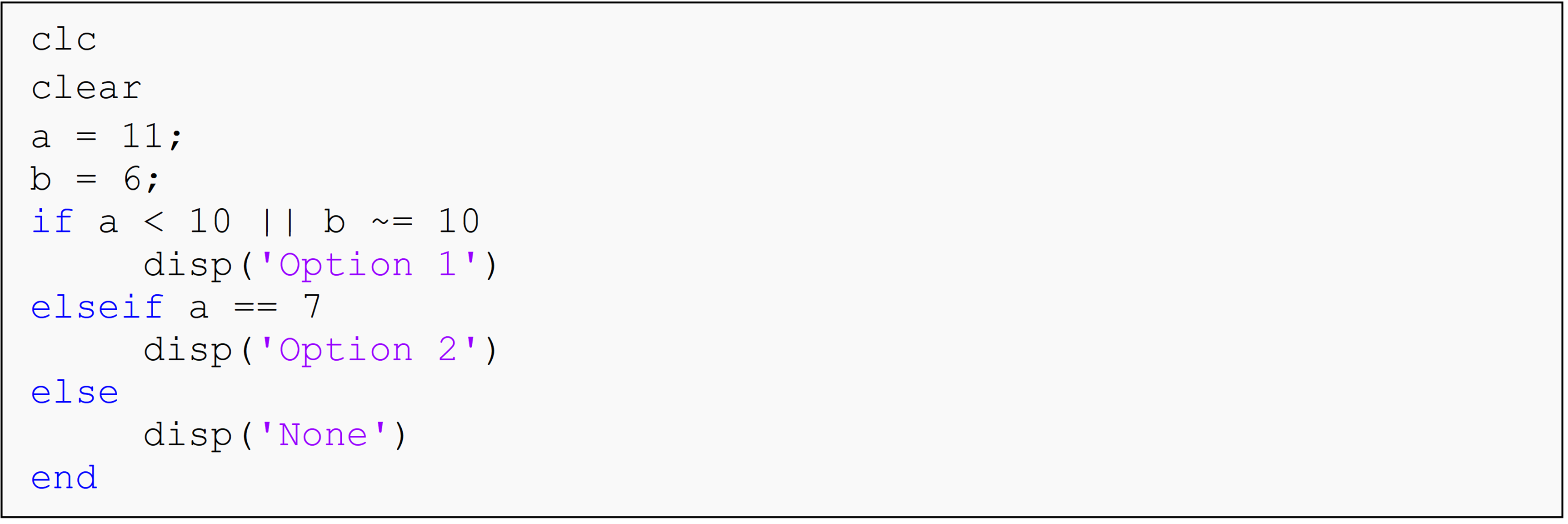(a)  Option 1

(b)  Option 2

(c)  None

(d)  The program displays nothing in the Command Window.

(5). Fill in the blank with one of the choices to make this code output b = 6.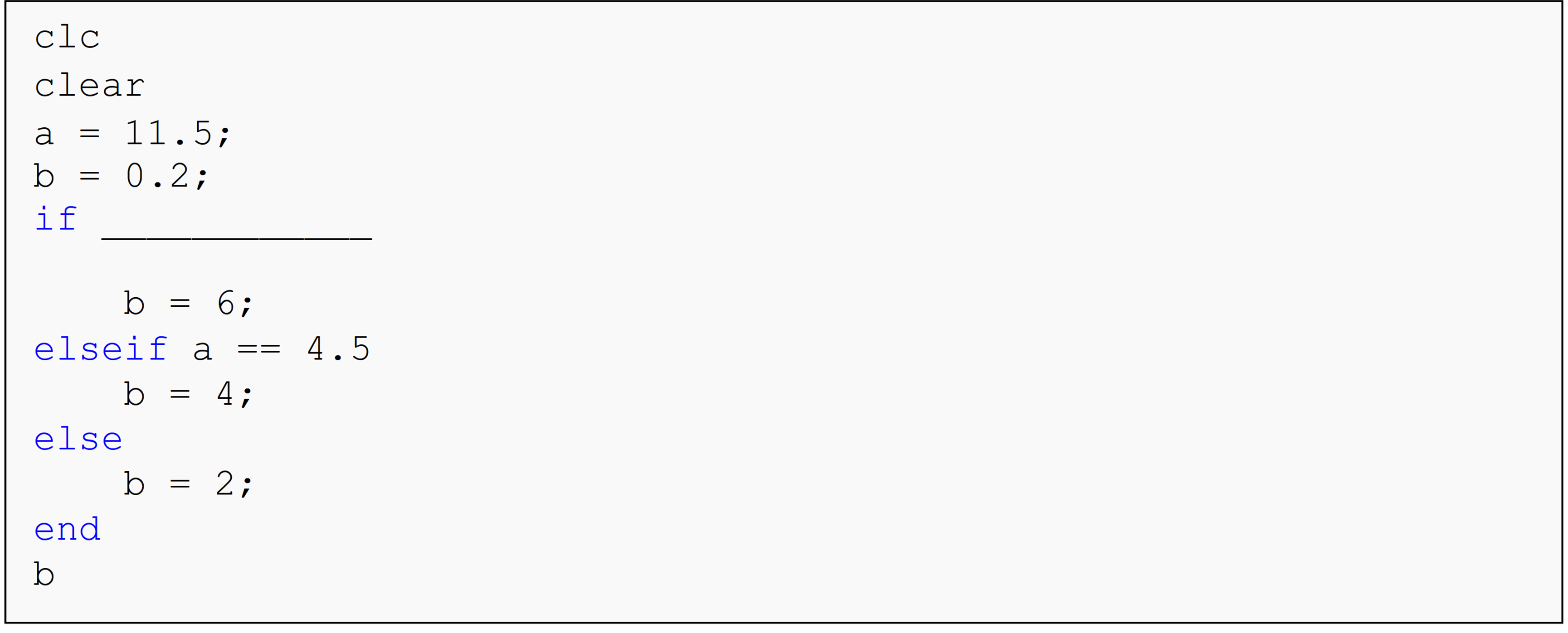(a)  a==b

(b)  a<=b

(c)  a~=b

(d)  a<b

## Problem Set

(1). Given an integer, write a MATLAB code for displaying whether the integer is a) positive (greater than zero), b) zero, c) or negative (less than zero). In Example 1 the solution is given using elseif, but here you are asked to use three separate if-end statements to complete the solution.

(2). A student’s grade is based on their score in four categories: homework, projects, tests, and final exam. The weight of each category and the student’s scores are given in Table A below.

Category Weight Example Scores
Homework 10% 88
Projects 12% 95
Tests 48% 76
Final exam 30% 91

The letter grades are given by

• $$90 \leq \text{A} \leq 100,$$

• $$80 \leq \text{B} < 90,$$

• $$70 \leq \text{C} < 80,$$

• $$60 \leq \text{D} < 70,$$ and

• $$\text{F} < 60$$

Write a program that calculates the overall percentage and letter grade for any student in the class and displays these in the Command Window. Use the given example as your test case.

(3). Your friend is having a hard time keeping track of their weekly schedule, which is as follows:

• Monday – Class at 7:30 AM and work at 5:00 PM

• Tuesday – Class at 2:00 PM and 4:30 PM

• Wednesday – Weekly group progress meeting at 11:30 AM and no class

• Thursday – Same as Tuesday

• Friday – Same as Monday

Your friend uses MATLAB every day, and therefore you told him you would write a program to keep track of his daily events. Using your knowledge of conditional case-structure, write a program where the output is a string of characters containing the events of a single day (not the entire week of events). The program input will be an integer which corresponds to a day of the week, where 1 is for Monday, 2 is for Tuesday, etc.

Provide an error statement if a number other than 1,2,3,4 or 5 is entered. Test your program using an input of 4 (corresponding to Thursday).

(4). So, you want my phone number and need to know my BMI? How shallow can you be? In 1998, the federal government introduced the body mass index (BMI) to determine an ideal weight based on a person’s height. Body mass index is calculated as 703 times the weight in pounds divided by the square of the height in inches, the obtained number is then rounded off to the nearest whole number (Hint: 23.5 will be rounded to 24; 23.1 will be rounded to 23; 23.52 will be rounded to 24). The criterion for the weight category is given as follows:

• $$\text{BMI} < 19$$ - Underweight

• $$19 \leq \text{BMI} \leq 25$$ - Healthy weight

• $$25 < \text{BMI} \leq 30$$ - Overweight

• $$\text{BMI} > 30$$ - Obese

Develop a MATLAB program that outputs an integer based on the person’s input weight, w, and height, h. The integer 0, 1, 2, or 3, is the output, depending on if a person is underweight, healthy weight, overweight, or obese, respectively.

Develop a program where based on a person’s input weight, w, and height, h, it outputs a description of their health state and a target healthy weight (if needed). The description to the health state output is, “Underweight”, “Healthy weight”, “Overweight”, or, “Obese”, and the target weight is to be rounded to the whole integer. Use the fprintf() function to describe all inputs and outputs.

(5). United States citizens pay federal income tax, social security tax, and Medicare tax on their wages.

Federal Income Tax: According to the Internal Revenue Service, a single-status U.S. citizen will pay 2018 federal income taxes according to the following chart*.

10% on income between $0 and$9,525

12% on the income between $9,526 and$38,700; plus $952.50 22% on the income between$38,701 and $82,500; plus$4,453.50

24% on the income between $82,501 and$157,500; plus $14,089.50 32% on the income between$157,501 and $200,000; plus$32,089.50

35% on the income between $200,001 and$500,000; plus $45,689.50 37% on the income over$500,001; plus $150,689.50 Social Security tax: In 2018, this is 6.2% on earnings up to$128,400**

Medicare tax: In 2018, this is 1.45% on all earnings.** An additional 0.9% applies to individuals who earn over $200,000.*** Complete parts (a) and (b): 1. Construct a pseudo code for a MATLAB program to find a person’s total tax liability based on their income. Assume all income is taxable. 2. Assume all income is taxable and write a program that inputs 1. the person’s income, income, and then outputs the owed 1. federal tax, fedTax, 2. social security tax, ssTax, 3. medicare tax, medTax. 4. total taxes, totalTax. Display all of the inputs and outputs by using the fprintf() function, complete with an explanation in a reasonable format. Run the program with incomes of$32,000, $85,000 and$269,000.